# Representation of a Lie algebra

in a vector spaceA homomorphismof a Lie algebraover a fieldinto the algebra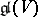of all linear transformations ofover. Two representations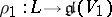andare called equivalent (or isomorphic) if there is an isomorphism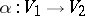for which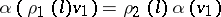for arbitrary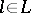,. A representationinis called finite-dimensional if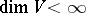, and irreducible if there are no subspaces in, distinct from the null subspace and all of, that are invariant under all operators,.

For given representationsand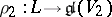one constructs the representations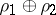(the direct sum) and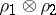(the tensor product) ofintoand, by putting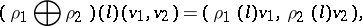where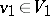,,. Ifis a representation ofin, then the formuladefines a representation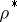ofin the space dual to; it is called the contragredient representation with respect to.

Every representation ofcan be uniquely extended to a representation of the universal enveloping algebra; this gives an isomorphism between the category of representations ofand the category of modules over. In particular, to a representationofcorresponds the ideal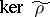in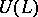— the kernel of the extension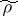. Ifis irreducible,is a primitive ideal. Conversely, every primitive ideal incan be obtained in this manner from an (in general, non-unique) irreducible representationof. The study of the space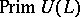of primitive ideals, endowed with the Jacobson topology, is an essential part of the representation theory of Lie algebras. It has been studied completely in caseis a finite-dimensional solvable algebra andis an algebraically closed field of characteristic zero (cf. ).

Finite-dimensional representations of finite-dimensional Lie algebras over an algebraically closed field of characteristic zero have been studied most extensively , , . When the field isor, these representations are in one-to-one correspondence with the analytic finite-dimensional representations of the corresponding simply-connected (complex or real) Lie group. In this case every representation of a solvable Lie algebra contains a one-dimensional invariant subspace (cf. Lie theorem). Any representation of a semi-simple Lie algebra is totally reduced, i.e. is isomorphic to a direct sum of irreducible representations. The irreducible representations of a semi-simple Lie algebra have been completely classified: the classes of isomorphic representations correspond one-to-one to the dominant weights; here, a weight, i.e. an element of the dual spaceof a Cartan subalgebraof, is called dominant if its values on a canonical basis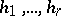ofare non-negative integers (cf. Cartan theorem on the highest weight vector). For a description of the structure of an irreducible representation by its corresponding dominant weight (its highest weight) see Multiplicity of a weight; Character formula.

An arbitrary element (not necessarily a dominant weight)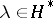also determines an irreducible linear representation of a semi-simple Lie algebrawith highest weight. This representation is, however, infinite-dimensional (cf. Representation with a highest weight vector). The corresponding-modules are called Verma modules (cf. ). A complete classification of the irreducible infinite-dimensional representations of semi-simple Lie algebras has not yet been obtained (1991).

Ifis an algebraically closed field of characteristic, then irreducible representations of a finite-dimensional Lie algebraare always finite-dimensional and their dimensions are bounded by a constant depending on. If the algebrahas a-structure (cf. Lie-algebra), then the constant is, whereis the minimum possible dimension of an annihilator of a linear form onin the co-adjoint representation . The following construction is used for the description of the set of irreducible representations in this case. Let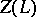be the centre ofand let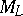be the affine algebraic variety (of dimension) whose algebra of regular functions coincides with(a Zassenhaus variety). The mapping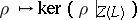makes it possible to assign a point on the Zassenhaus variety to each irreducible representation. The mapping thus obtained is surjective, the pre-image of any point ofis finite and for the points of an open everywhere-dense subset this pre-image consists of one element . A complete description of all irreducible representations has been obtained for nilpotent Lie algebras (cf. ) and certain individual examples (cf. , ). Most varied results have also been obtained for special types of representations.